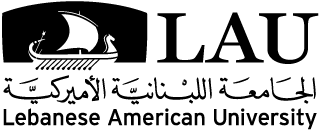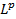# Courses

Back to All Disciplines

## Applied and Computational Mathematics Courses

### ACM701 Measure Theory and Functional Analysis

#### [3–0, 3 cr.]

The topics covered in this course are from measure theory and functional analysis. They include:  Measure spaces, measurable functions, integration, convergence of integrals,-spaces, Hilbert spaces (e.g, Riesz representation theorem, orthonormal bases, etc.), Banach spaces (e.g, dual spaces, open mapping theorem, Hahn-Banach theorem, etc.), functions of bounded variation and absolutely continuous functions.

### ACM702 Ordinary and Partial Differential Equations

#### [3–0, 3 cr.]

Topics in ODEs are: Linear systems (exponential of operators, diagonalization, Jordan forms, stability theory, etc.), non-linear systems (existence and uniqueness theorem, continuous dependence on initial conditions and parameters, linearization, stability and Lyapunov functions, etc.), and boundary-value problems. Topics in PDEs include: Boundary and initial-value problems, types of PDEs, well-posed and ill-posed problems, the Sturm-Liouville theory, Green’s functions, solutions of parabolic, elliptic, and hyperbolic equations.

### ACM710 Scientific Computing

#### [3–0, 3 cr.]

Fundamental techniques in scientific computing are covered: Floating-point representation and errors, locating roots of equations, interpolation and numerical differentiation, numerical integration and Gaussian quadrature formulas, systems of linear systems, Gaussian elimination with Scaled partial pivoting, tridiagonal and banded systems, matrix factorizations, iterative solutions, approximation by spline functions, Monte Carlo methods and simulation,  numerical solutions of ordinary differential equations, forward and backward Euler, predictor-corrector and Runge-Kutta schemes, shooting methods, and stiff ODE’s, minimization of functions.

### ACM711 Numerical Methods for Partial Differential Equations

#### [3–0, 3 cr.]

This course covers numerical methods for solving parabolic, elliptic, and hyperbolic partial differential equations with analysis of their accuracy, convergence, and stability. Topics include discrete Fourier transform, Lax’s equivalence theorem, Von Neumann’s stability condition, the method of characteristics, finite difference methods, Courant-Friedrichs-Lewy condition, TVD schemes, weak solutions and finite volume methods for hyperbolic systems, Godunov’s method, shock capturing methods, local linearization and Roe matrices, higher order methods. Prerequisite: ACM702.

### ACM712 Finite Element Methods

#### [3–0, 3 cr.]

Variational formulations of linear and nonlinear elliptic boundary value problems, eigenvalue problems, one-dimensional and multidimensional finite element methods, Galerkin’s method and extremal principles, piecewise Lagrange approximation, hierarchical bases, interpolation errors, function spaces and approximation Lagrange bases on triangles and rectangles, mesh generation and assembly, coordinate transformations, generation of element matrices and their assembly, discretization errors, adaptivity h- p- and hp-Refinement, semi discrete Galerkin methods, the Method of Lines, finite element methods in time, convergence and stability, error estimates.

Note: This course has not been taught since Fall 2020 and will not be taught in the academic year 2022-2023.

### ACM713 Numerical Linear Algebra

#### [3–0, 3 cr.]

Fundamentals of linear algebra, norms, singular value decomposition, projectors, QR factorization, Gram-Schmidt orthogonalization, Householder triangularization, conditioning, stability, conditioning of least squares problems, stability of least squares algorithms, Gaussian elimination, pivoting, stability of Gaussian elimination, Cholesky factorization, eigenvalue problems, reduction to Hessenberg or tridiagonal form, Rayleigh quotient, inverse iteration, QR algorithm with and without shifts.

### ACM714 Parallel Programming

#### [3–0, 3 cr.]

This course covers parallel computing, using groups of computers to solve problems at a greater computational speed. Topics include parallel computing techniques and algorithms, including divide and conquer, pipelined computations, genetic algorithms and simulated annealing. Topics also include synchronous and asynchronous computations, load balancing, shared memory, distributed memory, and distributed shared memory. Use of the message passing method of parallel computing, and use the standard parallel computing tools such as PVM and MPI.

Note: This course has not been taught since Fall 2020 and will not be taught in the academic year 2022-2023.

### ACM715 Mathematical Biology

#### [3–0, 3 cr.]

Topics covered are: A list of applications on special biological and medical topics will be done. This list includes the cardiovascular diseases: inflammatory reactions, biological flows and fluid structure interaction; cancer and its therapies including the circadian rhythms and the cell cycle. This may also incorporate the use of delay equations along with the stochastic modeling. The use of a specific tool/software (Matlab, Comsol Multiphysics) to implement and solve a discretized problem will be also treated.

Note: This course has not been taught since Fall 2020 and will not be taught in the academic year 2022-2023.

### ACM720 Convex Analysis and Optimization

#### [3–0, 3 cr.]

Topics covered are: Convex sets and functions, global and local minima, duality theory, saddle points and minima theory, subgradients and subdifferentials, optimality conditions, Lagrange multipliers, conjugate duality.

### ACM721 Nonsmooth Analysis and Applications

#### [3–0, 3 cr.]

Topics covered are: Proximal analysis in Hilbert space (Proximal normals, subgradients, distance functions, etc.), generalized gradients in Banach space (basic calculus rules, relationship to Proximal analysis, Bouligand tangent cone, etc.), constrained optimization and value functions, application in control theory (nonsmooth feedback theory). Prerequisites: ACM701 and ACM720.

### ACM722 Nonsmooth Optimal Control

#### [3–0, 3 cr.]

Topics covered are: Linear and non-linear control systems (controllability and observability, attainable sets, stability, linearization, etc.), linear and non-linear optimal control (smooth and non-smooth Pontryagin maximum principle, value function Hamilton-Jacobi theory, minimal time problem, existence theory, etc.). Prerequisites: ACM701, ACM702 and ACM 721.

Note: This course has not been taught since Fall 2020 and will not be taught in the academic year 2022-2023.

### ACM723 Calculus of Variations

#### [3–0, 3 cr.]

Topics covered are: Necessary conditions (Euler-Lagrange equations, Weierstrass condition, Jacobi equation, conjugate point theory, etc.), sufficient conditions (field of extremals, conjugate point theory, convex Largangians, etc.), Hamiltonian equations (least action principle, canonical transformations, Noether theorem, etc.). Prerequisite: ACM701.

Note: This course has not been taught since Fall 2020 and will not be taught in the academic year 2022-2023.

### ACM724 Linear Programming

#### [3–0, 3 cr.]

Topics covered are: Simplex method, degeneracy, cycling, fundamental theorem of linear programming, weak and strong duality theorems, dual simplex method, resource allocation, sensitivity analysis, simplex method in matrix notation, parametric analysis, and applications.

Note: This course has not been taught since Fall 2020 and will not be taught in the academic year 2022-2023.

### ACM725 Non-Linear Programming

#### [3–0, 3 cr.]

Topics covered are: Unconstrained optimization (local min and max, algorithms, Newton’s method, etc.), constrained optimization (first and second order optimality conditions, constraint qualifications, sequential quadratic programming, complementarity problem, penalty barrier, augmented Lagrangian methods,  interior point methods, etc.).

Note: This course has not been taught since Fall 2020 and will not be taught in the academic year 2022-2023.

### ACM726 Integer Programming

#### [3–0, 3 cr.]

Topics covered are: Polyhedral theory, valid inequalities, branch-and-bound algorithm and its variants, Integral polyhedral, IP duality. Prerequisite: ACM724 Linear Programming.

Note: This course has not been taught since Fall 2020 and will not be taught in the academic year 2022-2023.

### ACM727 Mathematical Methods for Applied Economics

#### [3–0, 3 cr.]

The course will cover mathematical methods for modeling problems of applied economics. The course will cover difference and differential equations, non-linear programming, dynamic programming, and optimal control theory.

### ACM788 Advanced Topics in Applied and Computational Mathematics

#### [3–0, 3 cr.]

(May be repeated)

Note: This course has not been taught since Fall 2020 and will not be taught in the academic year 2022-2023.